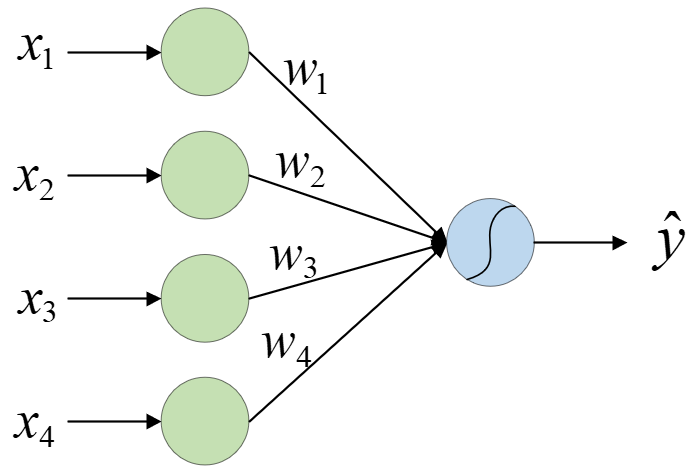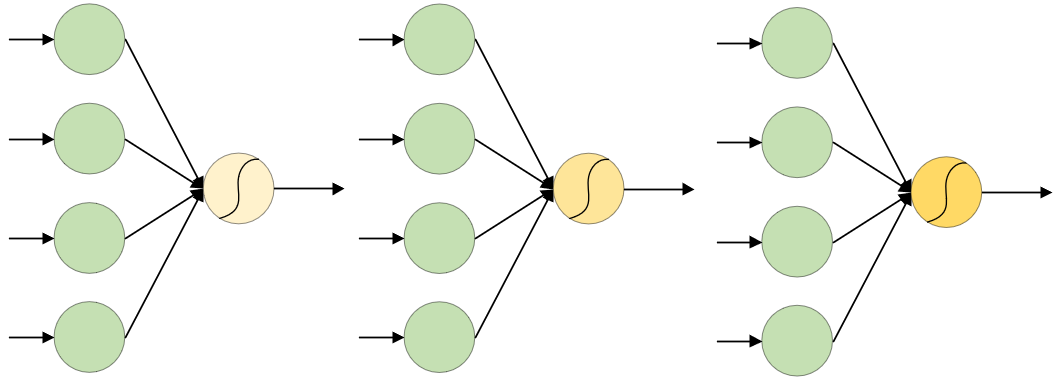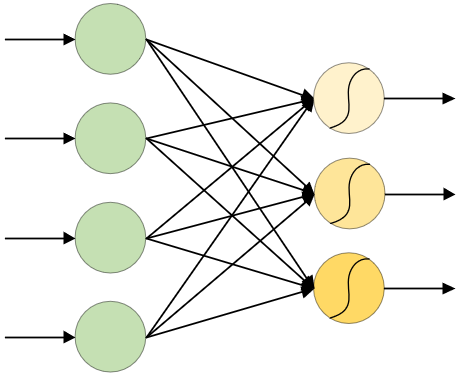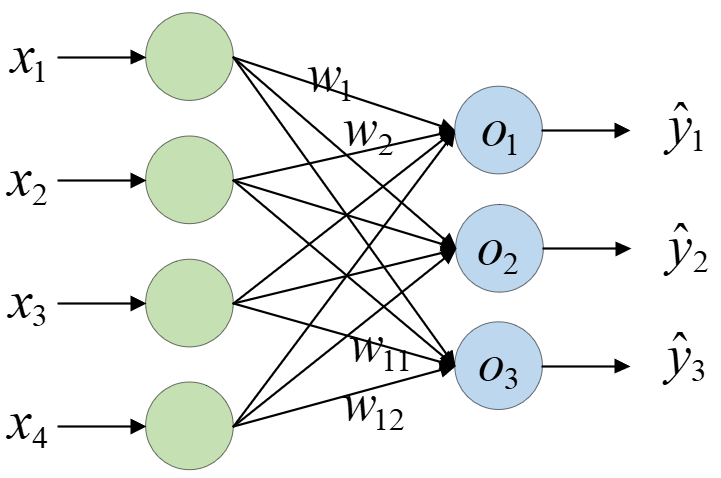# 想明白多分类，必须得谈逻辑回归

## 1 引例

《跟我一起机器学》 中，我们已经介绍了什么是逻辑回归回归。但是为了能更好的与深度学习相关内容进行衔接，在本篇文章中我们依旧是首先回顾一下逻辑回归，然后再一步步引入多分类直至过渡到深度神经网络的相关学习中。

### 1.1 从逻辑回归说起### 1.2 问如何解决多分类问题## 2 从单分类到多分类### 2.1 softmax回归o 1 = x 1 w 1 + x 2 w 2 + x 3 w 3 + x 4 w 4 + b 1 o 2 = x 1 w 5 + x 2 w 6 + x 3 w 7 + x 4 w 8 + b 2 o 3 = x 1 w 9 + x 2 w 10 + x 3 w 11 + x 4 w 12 + b 3 (1) \begin{aligned} o_1&=x_1w_1+x_2w_2+x_3w_3+x_4w_4+b_1\\[1ex] o_2&=x_1w_5+x_2w_6+x_3w_7+x_4w_8+b_2\\[1ex] o_3&=x_1w_9+x_2w_{10}+x_3w_{11}+x_4w_{12}+b_3\\[1ex] \end{aligned}\tag{1}

y ^ 1 , y ^ 2 , y ^ 3 = S o f t m a x ( o 1 , o 2 , o 3 ) (2) \hat{y}_1,\hat{y}_2,\hat{y}_3=Softmax(o_1,o_2,o_3)\tag{2}

y ^ 1 = exp ⁡ ( o 1 ) ∑ i = 1 3 exp ⁡ ( o i ) ,      y ^ 2 = exp ⁡ ( o 2 ) ∑ i = 1 3 exp ⁡ ( o i ) ,      y ^ 3 = exp ⁡ ( o 3 ) ∑ i = 1 3 exp ⁡ ( o i ) (3) \begin{aligned} \hat{y}_1=\frac{\exp{(o_1)}}{\sum_{i=1}^3\exp{(o_i)}},\;\; \hat{y}_2=\frac{\exp{(o_2)}}{\sum_{i=1}^3\exp{(o_i)}},\;\; \hat{y}_3=\frac{\exp{(o_3)}}{\sum_{i=1}^3\exp{(o_i)}} \end{aligned}\tag{3}

### 2.2 交叉熵损失函数

H ( y ^ ( i ) , y ( i ) ) = − ∑ j = 1 q y j ( i ) log ⁡ y ^ j ( i ) (4) H(\hat{y}^{(i)},y^{(i)})=-\sum_{j=1}^qy^{(i)}_j\log{\hat{y}^{(i)}_j}\tag{4}

H 1 = − ( 0 ⋅ log ⁡ 0.3 + 0 ⋅ log ⁡ 0.1 + 1 ⋅ log ⁡ 0.6 ) = 0.51 H 2 = − ( 0 ⋅ log ⁡ 0.7 + 0 ⋅ log ⁡ 0.2 + 1 ⋅ log ⁡ 0.1 ) = 2.3 (5) \begin{aligned} H_1&=-(0\cdot\log{0.3}+0\cdot\log{0.1}+1\cdot\log{0.6})=0.51\\[1ex] H_2&=-(0\cdot\log{0.7}+0\cdot\log{0.2}+1\cdot\log{0.1})=2.3\\ \end{aligned}\tag{5}

L ( Θ ) = 1 m ∑ i = 1 n H ( y ^ ( i ) , y ( i ) ) (6) \mathcal{L}(\Theta)=\frac{1}{m}\sum_{i=1}^nH(\hat{y}^{(i)},y^{(i)})\tag{6}

## 3 总结

### 引用

动手深度学习

示例代码：https://github.com/moon-hotel/DeepLearningWithMe

### 推荐阅读

Pytorch之Linear与MSELoss

Pytorch之拟合正弦函数你会吗？

你告诉我什么是深度学习12-011万+05-17510
11-114666
05-251万+
04-144万+
02-037129
08-093513
03-023154
09-031万+
01-084万+
08-131万+
09-2843万+
02-231万+
11-122万+
07-042万+
01-196934
04-033万+
11-103418